﻿ Pauli exclusion principle in QFT spin

# Pauli exclusion principle = force ?

## Pauli exclusion principle is NOT a force ?

### [ Four fundamental forces don't include Pauli principle. ]

(Fig.1)  Only Coulomb and gravity are realistic forces in these ↓.Quantum mechanical Pauli exclusion principle is unrealistic and inconsisten with the fact.

The present particle physics claims there are four fundamental forces such as Coulomb, strong, weak and gravity forces.

According to Pauli exclusion principle, No two electrons can simultaneously occupy the same quantum state with respect to orbital and spin.

So the 3rd electron in lihium is expelled from inner 1s orbital into outer 2s orbital, resisting Coulomb attraction between the electron and nuclues.

This Pauli exclusion force is such a strong repulsion that the nucleus cannot pull the electron closer to it.  This Pauli repulsion isn't considerted to be a force.

Even Pauli himself was unable to give a logical reason for the exclusion principle ( this p.6 ).  Electron spin-spin magnetic interaction is too weak to cause this strong repulsion.

## Quantum mechanical model is unrealistic.

### [ Angular momentum zero + faster-than-light spin = quantum. ]

(Fig.2)  Quantum mechanics gives unreal hydrogen model with spin ↓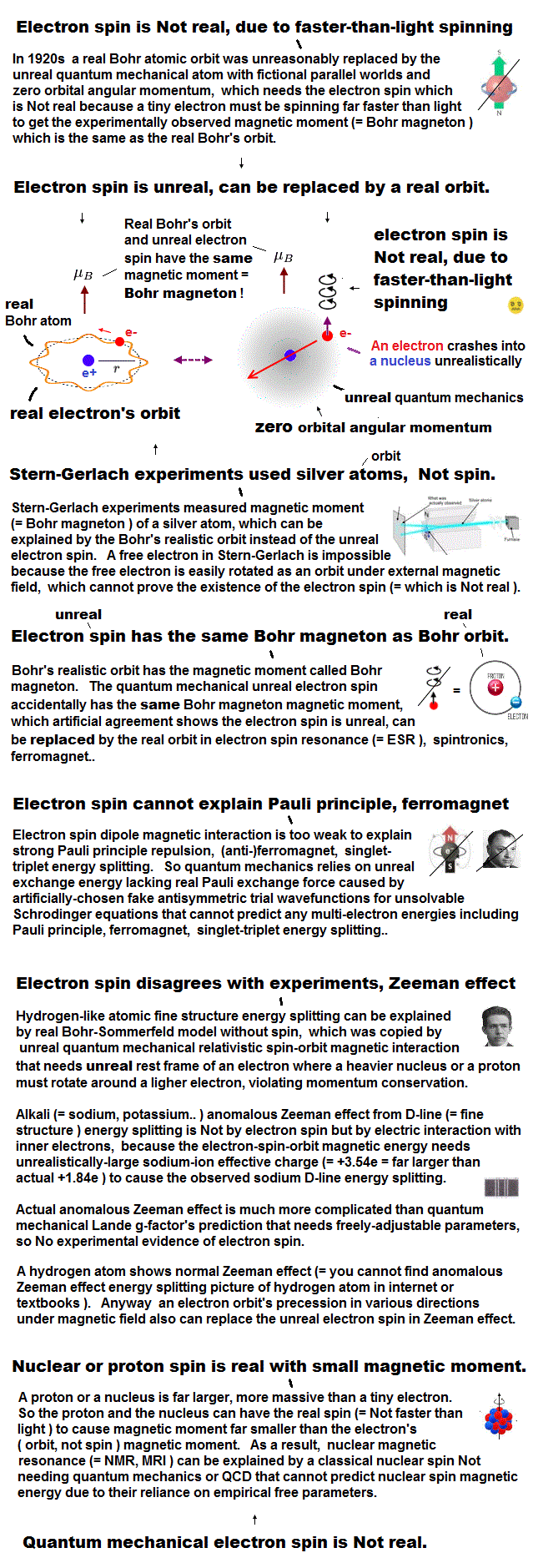Bohr model first succeeded in explaining hydrogen energy levels, and was replaced by quantum mechanics in 1920s.

Surprisingly, quantum mechanics requires that s orbitals (= ground state ) have zero angular momentum ( this p.2 ).  ←Electrons often hit nucleus ?

Magnetic moment of hydrogen atom is known to be equal to Bohr magneton, which was first predicted by Bohr model.

But the quantum mechanical hydrogen with zero angular momentum cannot generate the magnetic moment.

This is the reason why they fabricated artificial spin, which cannot be spinning realistically.

## Electron spinning far exceeds light speed !

### [ Spinning speed of "point"-like electron is much faster than light ! ]

(Fig.3)  Point-like electron ( radius r → 0 ), rotation v → ∞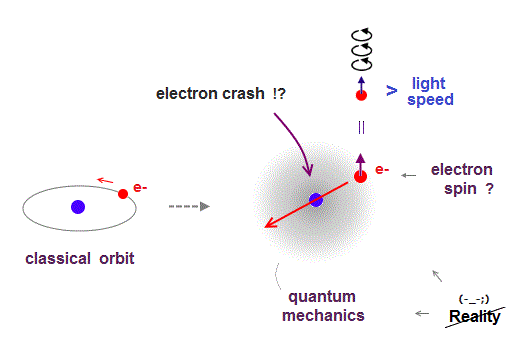Angular momentum is given by mv × r ( v = velocity, r = radius ).
Electron spin also has angular momentum 1/2ħ, they claim

The problem is an electron is very tiny, point-like.
The point-like particle means its radius r is almost zero.

So to get the angular momentum 1/2ħ, the electron spinning must far exceed light speed ( this p.5, this )

So the electron spin lacks reality.
Even Pauli ridiculed the idea of "spinning electron".

But in "s" orbital of Schrodinger's hydrogen, this electron spin is the only generator of magnetic moment.

So they had no choice but to accept this strange spin ( Not as real spinning and speed ).

## Spin has the same Bohr magneton.

### [ Electron spin has the same magnetic moment as Bohr's orbit ! ]

(Fig.4)  ↓ Lucky coincidence ?  Same magnetic moment.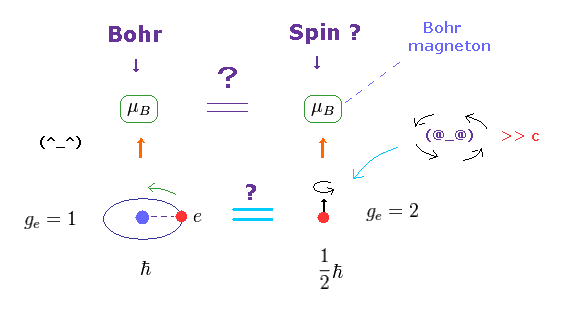It's known that hydrogen atom has magnetism equal to Bohr magneton, which can be explained by Bohr's classical orbit and de Broglie theory.

After quantum mechanics was born, its Schrodinger wavefunction has No orbital angular momentum to explain this magnetism.

So the physicists at the time invented strange spin, and they artificially defined the spin's magnetic moment as the same Bohr magneton !

This is a very far-fetched interpretation.
Spin's angular momentum is 1/2ħ, which is half of Bohr's ħ angular momentum.

So they decided that spin g-factor is twice (= 2 ) the Bohr's orbit (= 1 ).
"g-factor" means the ratio of magnetic moment to angular momentum.

As a result, they claim spin can also has the same Bohr magneton.
We can only measure the magnetism, neither angular momentum nor g-factor.

The problem is there is No physical reason why "spin" cannot stop and it has the same Bohr magneton.

## Stern-Gerlach experiment shows spin is a magnet ?

### [ Spin magnetic moment is equal to Bohr magneton (= μB ). ]

In Stern-Gerlach experiment, they got some atoms through magnetic field to measure the magnitude of magnetic moment of each electron.

Surprisingly, the experiments showed that the magnitude of magnetic moment of electron spin is just equal to Bohr magneton in Bohr's classical orbit ( this p.4 )

We can safely say Stern-Gerlach experiment proved the magnetic property in Bohr's orbit ( Not unreaslitic spin ! )

## Spin-spin magnetic interaction is too weak !

### [ Spin cannot explain strong Pauli principle ! ]

(Fig.6)  Spin magnetic energy= 0.001 eV vs Pauli repulstion = 30 eV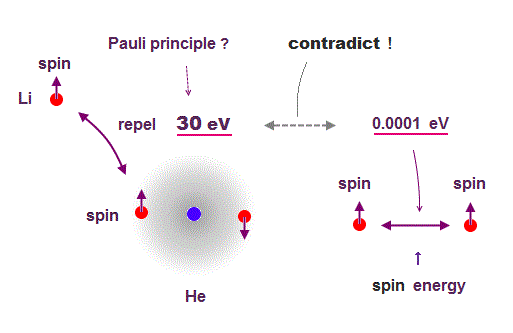Stern-Gerlach experiment showed electron spin has the magnetism equal to Bohr magneton.

From this measured magnetic moment, we can get the magnetic interaction between two electron spins.

This spin-spin magnetic energy is very weak (= about 0.001 eV ), compared with repulsive energy required for Pauli exclusion principle (= 30 eV ).

It means spin magnetic moment has nothing to do with Pauli exclusion principle, and they created artificial concept such as "exchange force" ( this p.7, this p.6 ).

After finding the magnitude of electron spin disagreed with strong Pauli principle, they fabricated artificial new force (= exchange ? ).  ← this is NOT a science !

## Electron spin failed in Pauli exclusion principle.

### [ Spin cannot explain why Li 3rd electron is expelled. ]

(Fig.7)  Expelling lithium 3rd electron to outer 2s needs 30 eV repulsion !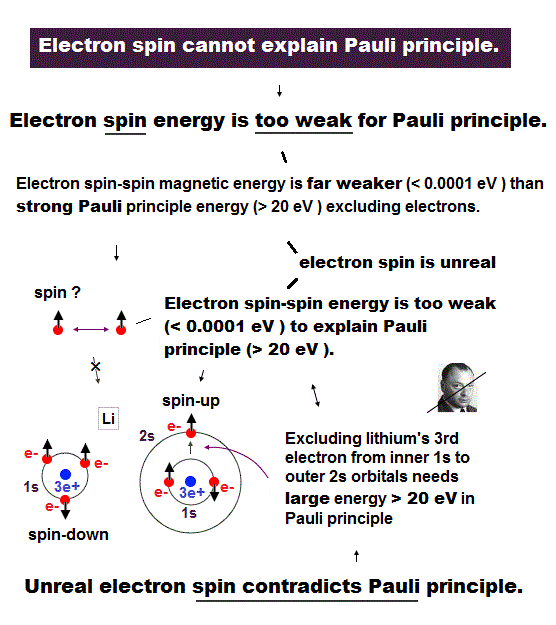Pauli exclusion principle claims that 3rd electron of lithium (= Li ) must be expelled from outer 2s orbital, resisting Coulomb attraction from positive nucleus.

This Pauli repulsive force must be very strong (= about 30 eV in Li ), so weak spin-spin magnetic interaction cannot explain it.

This means electron spin model failed in strong Pauli principle, and we have to find other plausible mechanisms as this replusion.

Spin-spin magnetic energy is very weak, 0.0001 eV when their distance is 1 Å.  See calculation.

When even 3rd electron of Li enters inner 1s orbital, its total energy becomes -8.46 Hartree (= -230 eV ), which is 30 eV lower than the actual energy.

## Quantum field theory explains Pauli principle ?

### [ The present physics cannot specify real reason of Pauli exclusion ! ]

(Fig.8)  Anticommuting of nonphysical operators = Pauli exclusion ?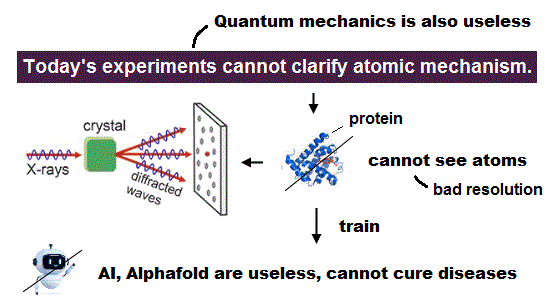It is said that "force" of Pauli exclusion prinicple can be explained by quantum field theory.  But this is misleading.

Because the quantum field theory is too abstract and too far away from our actual world to specify true reason for Pauli exclusion principle.

So the present physics cannot tell you what force is involved in strong Pauli exclusion principle !

## Dirac equation tells us Pauli exclusion principle ?

### [ Einstein relation → Dirac equation with spin ? ]

(Fig.9)  Dirac equation explains Pauli exclusion principle ?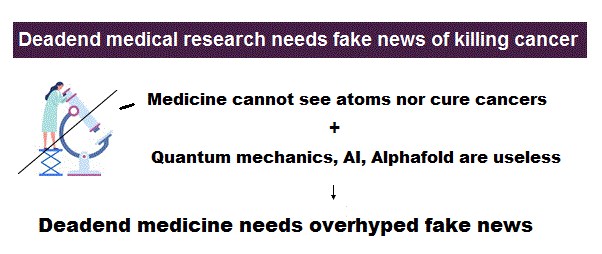Dirac equation is the most important one which forms the basis of all quantum field theories.  The problem is its form is out of touch with reality.

And the present physics claims that this Dirac equation tells us why Pauli exclusion principle occuurs.  But in fact, it doesn't.

Relativistic Dirac equation was gotten by dividing Einstein quadratic relation into linear functions.

In compensation for linear function, Dirac equation must contain 4 × 4 gamma (= γ ) matrices, which consist of spin Pauli matrices (= σ ).

This is the reason they claim Dirac equation succeeded in combining "spin" and relativity.  But "σ = spin ?" is just artificial definition with No grounds.

## An electron in quantum field theory is nonsense.

### [ Symbol "c" represents an electron ? ]

(Fig.10)  Quantum field theory cannot describe a concrete electron.All quantum field theories are based on relativistic Dirac equation.
How is each electron described in this quantum field theory ?

The problem is these quantum field theories have No ability to describe concrete figure and motion of each particle.

All it can show is an abstract and simple symbol "c" as an electron.
And anticommutation relation represents Pauli exclusion force ( this p.3 ) ?

## Electron = meaningless math symbol ?

### [ Quantum field theory cannot describe a true electron ! ]

(Fig.11)  It can do only two simple actions: create and vanish !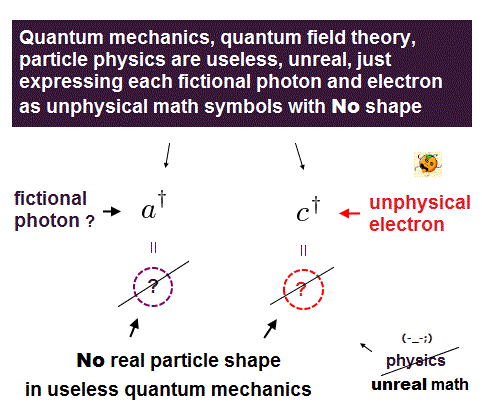Any quantum field theories cannot describe actual electrons.
It only shows a single letter (= "c ") as an electron.

Using this single letter, all quantum field theory can do is two actions: create and annihilate each electron !

When you see an electron ( here "a" ) and a photon, you will find the quantum field theory is valueless and useless for us.

This unrealistic quantum field theory makes the present condensed-matter physics useless using fictional photon.

## Pauli exclusion principle ?

### [ Pauli exclusion by quantum field theory is nonsense. ]

(Fig.12)  Pauli exclusion principle = anticommutation of two symbols ?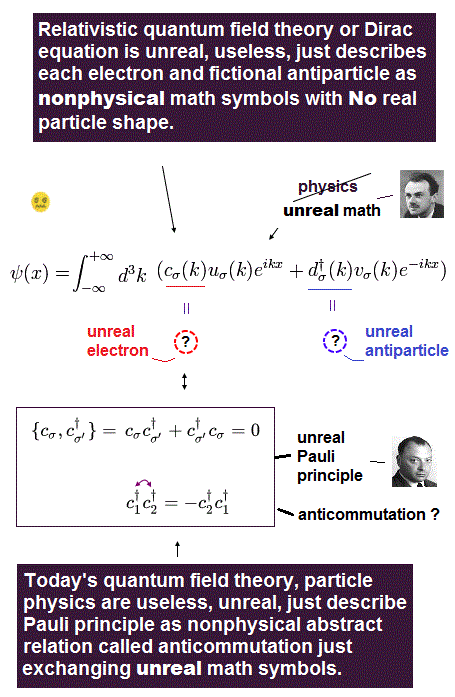Pauli exclusion principle claims two electrons cannot be in the same kind of state in orbital and spin, though spin is too weak to cause this.

It is said relativistic quantum field theory can explain why this Pauli exclusion principle occurs.  But it is a big lie.

All quantum field theory can show is anticommutaton of two electrons' symbols.  That's all.  No more detailed is mentioned.

If two electrons are in the same state ( c1 = c2 ), these c must be zero, when exchanging them reverses the whole sign (= anticommutation ).

So c1 and c2 must be different states from each other.  No more detailed mechanism in Pauli principle is mentioned in quantum field theory !

Quantum field theory does NOT try to clarify further mechanism in Pauli exclusion than this meaningless exchange relation.

## Electron spin in metal is unreal.

### [ Spin is too weak to cause ferromagnet. ]

(Fig.13)  ↓ Spin model is broken at room temperature !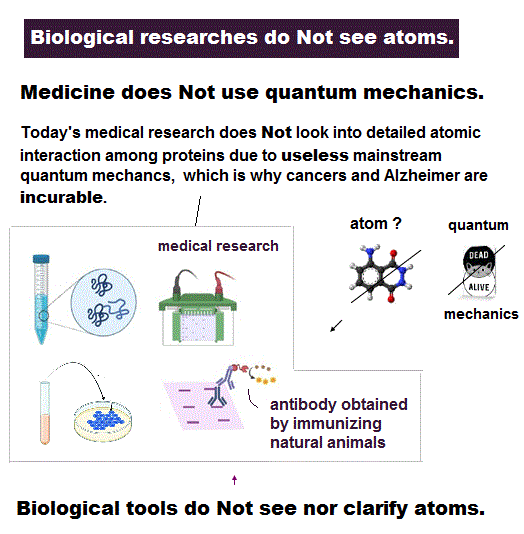Quantum mechanics claims that (anti)-ferromagnetism is caused by electron spin.  But this is a big lie.

The magnetic energy by electron spin is too weak to cause ferromagnet.
Spin-spin interaction is easily broken at low temperature ( ~ 0.3 K ).

But actual iron can keep ferromagnetic at 1043 K ( this p.7 ).

In fact, electron's spin disagrees with many experimental results !
"Spin" is used only as a "mark" with No physical meaning.

So we must give up contradictory spin model to investigate further mechanism !

It's natural to think that ferromagnet is maintained stably by Coulomb interaction among electrons' orbits ( not spin ! )

## Ferromagnet has nothing to do with spin.

### [ Spin magnetic moment is too weak to explain ferromagnetism. ]

(Fig.14)  Spin magnet is too weak to explain ferromagnet.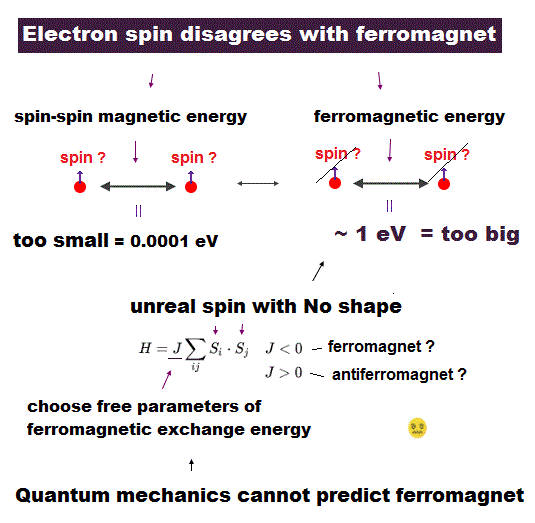You may think Spintronics and excitonics are useful (← ? ) for your career.

But almost nobody knows electron spin lacks reality !
Its spinning far exceeds light speed.

You may hear spin is tiny magnet with the magnitude of Bohr magneton.
But this is not true, and disagrees with experiment.

Spin-spin magnetic interaction is too weak to explain actual ferromagnet.  See this p.6 this p.7.  Spin can be replaced by more realistic model.

Then, what the heck does this spin model mean ?
It uses "Heisenberg" spin model ( this p.3 ).

But this Heisenberg spin model is too old, which was introduced in 1920s, and it's too abstract to describe actual phenomena ( this p.2 ).

This spin model just puts nonphysical symbols side by side.  So useless.
Parameter J is arbitrarily chosen.  J > 0 = antiferromagnet, J < 0 = ferromagnet.

## Electron de Broglie wave was confirmed.

### [ de Broglie relation was confimed in various experiments. ]

(Fig.15)  Double-slit, Davisson-Germer proved electron's de Broglie wavelength.In de Broglie relation, electron's wavelength λ is given by λ = h/mv, where m and v are electron's mass and velocity.

This important matter-wave relation was confirmed in various experiments such as doube-slit, Davisson-Germer and this.

So there is No room for doubt that this de Broglie wave is true.

In these experiments, an electron is driven out from destructive interference regions by the "force" of de Broglie wave ( this p.25 )

This strong "expelling force" by de Broglie wave interference can explain strong Pauli exclusion force !

## An integer times de Broglie wavelength is needed

### [ to avoid destructive interference of de Broglie wave. ]

(Fig.16)  Quantum orbit satisfies an integer times de Broglie wavelength.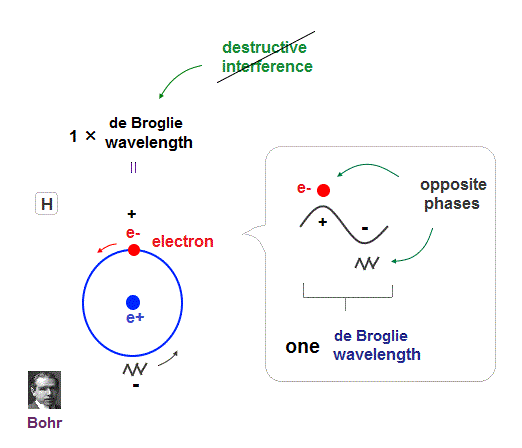Only Coulomb force is insufficient to explain why atomic energy levels are quantized, abd why an electron doesn't fall into nucleus.

Bohr model succeeded in getting actual atomic energies, proposing each orbit is an integer times de Broglie wavelength, as well as Schrodinger's hydrogen .

An integer multiple of de Broglie wavelength means an electron can avoid destructive interference and be stable.

Without this de Broglie wave, each electron can be attracted to positive nuclei, until they stick to each other and its energy is unlimitedly lower !

So the repulsive force by electron's de Broglie wave is strong enough to cause Pauli exclusion force.

## de Broglie wave interference explains helium.

### [ Why helium is stable and doesn't form compounds ]

(Fig.17) Two de Broglie waves cross perpendicularly = stable.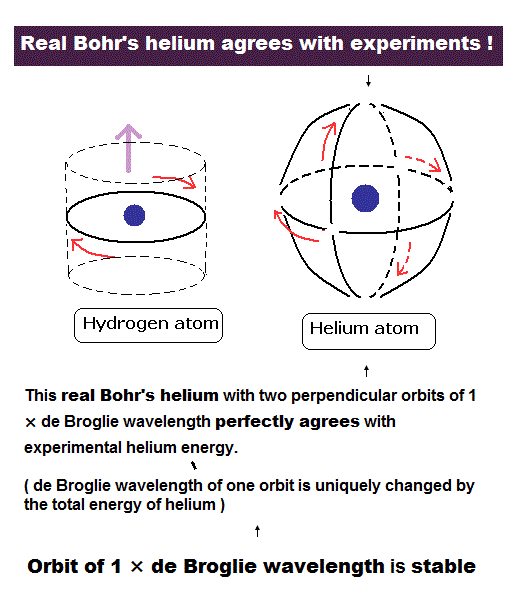If two 1 × de Broglie wavelength orbits are in the same plane in old Bohr's helium model, their opposite wave phases cause destructive interference and vanish.

To avoid vanishing de Broglie's wave, two electron orbits in actual helium must be perpendicular to each other.  Each orbit is one-de Broglie wavelength.

If the two orbits are perpendicular to each other, their wave phases are independent from each other and can be stable, not canceling each other.

This helium model considering actual de Broglie wave interference just agrees with experimental results of all atoms !

## Stable de Broglie waves determine helium structure.

(Fig.18) Old Bohr's helium = electrons are expelled.   New Bohr helium = stable.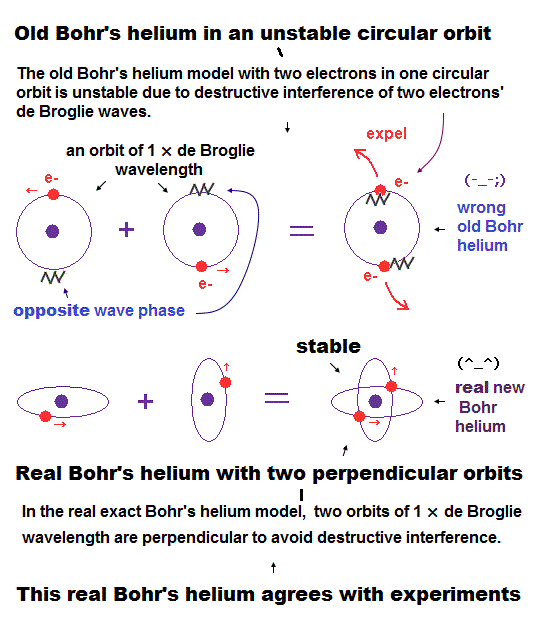In 1 × de Broglie wavelength orbit, the opposite sides of nucleus contain the opposite wave phases, which cancels another phase.

When two de Broglie waves are just perpendicular to each other, they can avoid destructive interference between these opposite phases.

There is NO more space for the third electron to enter this helium (= Pauli exclusion principle can be explained ).

We succeeded in expressing Pauli exclusion principle in all atoms using this de Broglie wavelength.

## New Bohr's helium can explain Pauli exclusion principle.

(Fig.19)  Pauli exclusion principle by de Brolgie wave interference.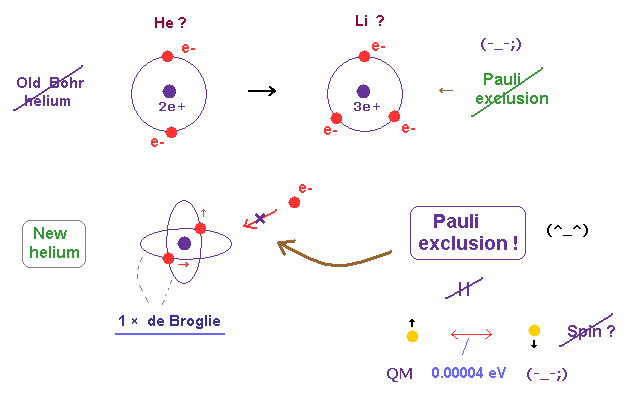There is NO space for the third electron to enter in this helium model based on de Broglie wave interference (= Pauli exclusion principle ).

Because, if the third electron enters the orbit of 1 × de Broglie wavelength in this new Bohr's helium, it cannot be perpendicular to both of two other waves.

On the other hand, in old Bohr helium, the third electron of Li can enter this orbit, because it does NOT depend on cancellation between de Broglie waves.

Spin-Spin magnetic dipole moment interactions are too week to explain strong Pauli exclusion principle.
For example, fine structure of hydrogen is ONLY 0.000045 eV. Spin-spin coupling is weaker than it.

As a result, Only de Broglie wave's interference is left for describing strong Pauli exclusion principle also in bonding number.

## de Broglie wave causes Pauli exclusion principle !

### [ Too weak spin cannot explain strong Pauli repulsion. ]

(Fig.20)  Only de Broglie wave interference can cause strong repulsion.Electron spin lacks reality, and its magnetic moment is too weak to generate strong repulsion in Pauli exclusion principle.

So there is only one thing left to cause this strong repulsion in lithium 3rd electron.  This is de Broglie wave interference !

Actually destructive interference in doube-slit experiment proved this de Broglie wave has power enough to expel each electron, and compatible with hydrogen.

## Conclusion:  Spin model failed in Pauli principle !

### [ So we have to consider other de Broglie wave interference. ]

(Fig.21)  to generate strong repulsion in Pauli exclusion principle.In conclusion, electron spin magnetic moment is too weak to kick out electrons from inner orbitals in Pauli exclusion principle.

Quantum fiel theory has NO ability to describe the reason for this Pauli exclusion in detail.  It just shows meaningless anticommutation of nonphysical operators.

From actual experiments and quantized hydrogen energy levels, we can conclude that de Broglie wave interference is the cause of strong Pauli exclusion principle.

## Quantum mechanics violates two basic postulates.

### [ Two postulates: Coulomb and de Broglie wave are violated in multi- electron atoms.]

(Fig.22)  Why Schrodinger equation cannot handle multi-electron atoms ?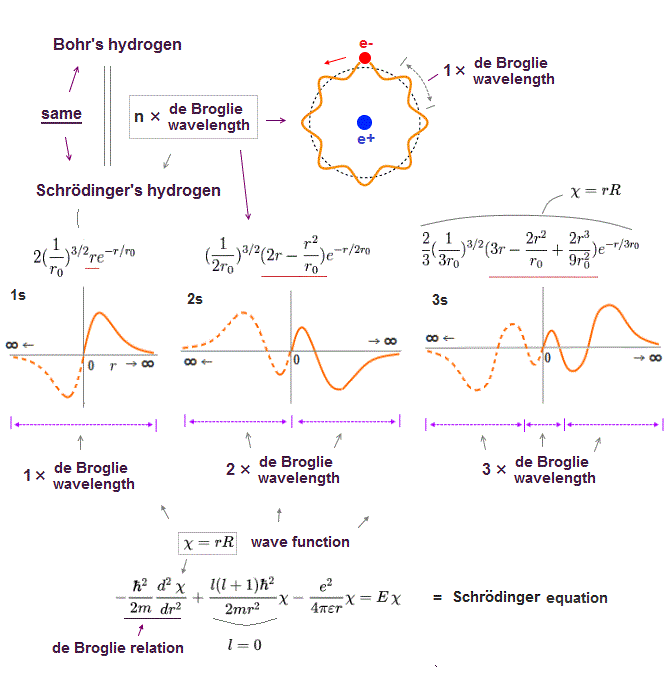Schrodinger equation is also based on two postulates such as Coulomb force and de Broglie wave relation.

In the only solvable one-electron hydrogen, total energy E is conserved as the single common value in any electron's positions (= Coulomb ).

And its solution naturally obeys an integer times de Broglie wavelength as boundary condition (= de Broglie ).

It is quite natural that we can predict any values even in multi-electron atoms obeying these basic common postulates.

The problem is quantum mechanics violates these two basic postulates in multi-electron atoms except hydrogen !

No solution in multi-electon atoms means we cannot find the single common energy value in any electrons' positions.

In the same way, No solution means the "integer times de Broglie wave rule" is ignored and violated.

So violating two basic postulates is the main reason quantum mechanics cannot predict any values in multi-electron atoms.

## Chosen wavefunction is Not a true orbital.

### [ Cannot solve → choose virtual function = not true energy ! ]

(Fig.23)  "Choose" trial functions → integral over all space.Here we explain why these "chosen" wavefunction cannot give true ground state energy of helium.

After choosing some trial wavefunction of unsolvable atoms, they integrate them over all space, and get, what they call, approximate total energy E'.

The point is this approximate energy E' is just an average energy in a collection of different energies depending on different electrons' position.

Originally, the sum of kinetic and potential energy in any electrons' positions must be equal to the single common ground state energy E.

But "unsolvable" multi-electron wavefunctions don't satisfy this basic condition.

So, this "average" energy E' does Not mean the single common ground state energy in any positions of helium.

## Quantum mechanics stops science !

### [ Experimental Data → fictional quasiparticle, effective mass ! ]

(Fig.24)  We must use experimental data to predict "real concept" !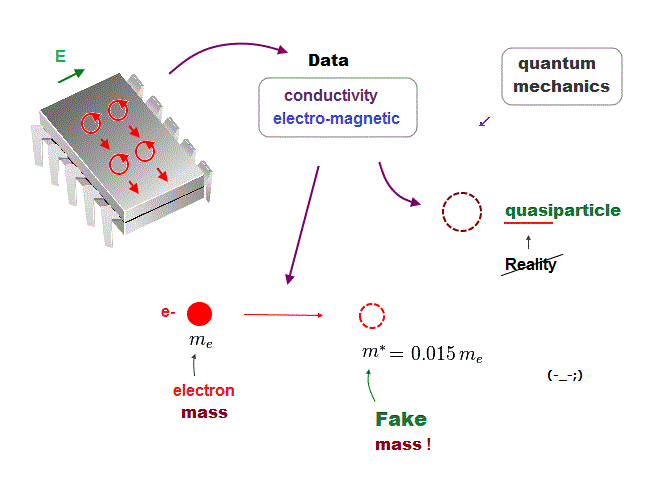In the present quantum mechanics, even when we get some new experimental data, we cannot use them to clarify real mechanisms !

Instead, quantum mechanics forces us to use these data to create artificial quasiparticle, effective mass ..  So our science stops its progress.

This is a very serious problem.  We must address it immediately not to increase more educational victims under false science.

## Only planetary model can keep two basic postulates.

### [ Two basic axioms: Coulomb and de Broglie wave are valid only in classical orbits. ]

(Fig.25)  Coulomb energy conservation and an integer times de Broglie wavelength.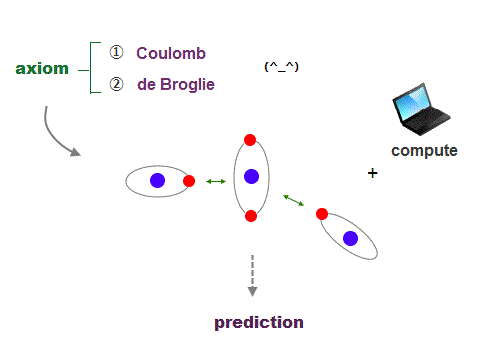It's quite natural that if we keep basic postulates, we can predict any physical values ( with the help of modern computer ).

These two postulates are Coulomb energy conservation ( in any electrons' positions ) and an integer times de Broglie wavelength generating Pauli force.

There is No way other than computing each Coulomb force and changing electrons' position in orthodox way to keep the single common ground state energy.

Of course, to obey an integer times de Broglie wave rule, this computing method using concrete orbits is the only one.

So the first thing for researchers to do is determine the more precise electronic motion model step by step, comparing experiments and the model's prediction.

I'm sure this realistic model based on two postulates will contribute to curing fatal diseases such as cancer, ALS, dementia someday.2016/4/15 updated. Feel free to link to this site.